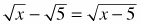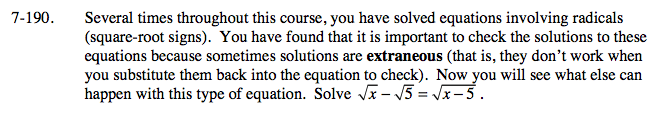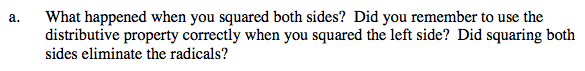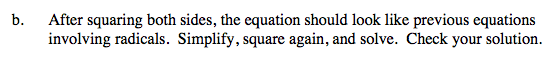Home > A2C > Chapter 7 > Lesson 7.3.3 > Problem7-190

7-190.
1. Several times throughout this course, you have solved equations involving radicals (square-root signs). You have found that it is important to check the solutions to these equations because sometimes solutions are extraneous (that is, they don't work when you substitute them back into the equation to check). Now you will see what else can happen with this type of equation. Solve. Homework Help ✎

1. What happened when you squared both sides? Did you remember to use the distributive property correctly when you squared the left side? Did squaring both sides eliminate the radicals?

2. After squaring both sides, the equation should look like previous equations involving radicals. Simplify, square again, and solve. Check your solution.Remember that there are two separate radicals on the left.x = 5